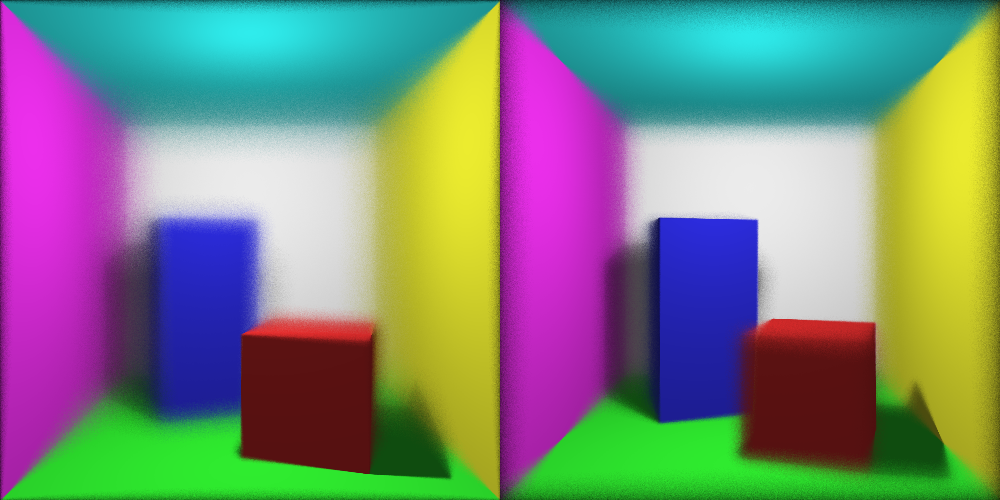## Depth of Field

11 May 2019

Until now we assumed our camera to be a perfect pinhole model: the convergence area is an ideal point. Both the eye and the camera have a mechanism to filter light, respectively the pupil and the aperture. In practice the pinhole is always a few millimeters wide, in order to capture enough light and reduce the required exposure time. The side effect is that all elements of the scene cannot appear crisp at the same time; typically only a single depth of field can be captured clear while the others are blurry. It’s essentially what is meant by the term “focus”.

We are able to reproduce this phenomenon in ray tracing, with once again a stochastic sampling process. We will introduce two additional parameters to our camera:

• $d_{focus}$ the focused distance in respect to the camera
• $r_{aperture}$ the radius of the aperture

And of course not to mention a sampling rate.

By the way we defined our parameters, we want object located at the focused distance to appear clear. Therefore for each pixel we will compute the point that would come out the sharpest if there happened to be an object there.The aperture is the left black box, the chosen focus is represented by the dashed line and the three objects represented by three colored circles. Observe that all the rays intersect on the arc, and that the only object that will render correctly is the blue one.

Let $F$ be our focal point (the position of the camera) and $\vec{r}$ the normalized ray for that pixel. We have $P := F + d_{focus} \cdot \vec{r}$, the focused point. Now let’s define two variables, $\theta \in [0, 2 \pi]$ and $\rho \in [0, r_{aperture}]$. Together they will allow us to determine the new focal point:

The last step is to sample rays passing by $P$ and different focal points on the aperture plane:Focusing respectively the red and the blue cuboid with 100 samples per pixel